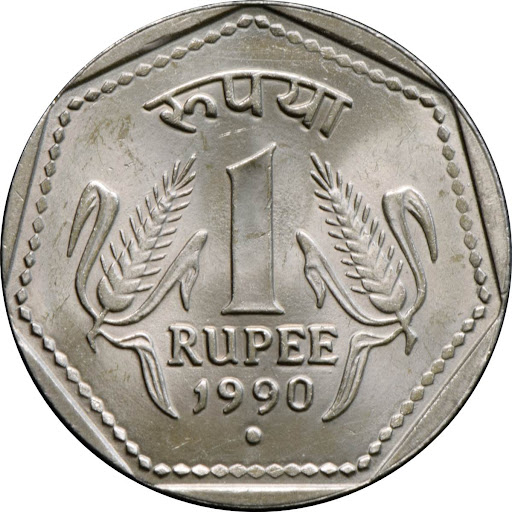# Bsnl Academy MCQ on Measurement Pdf

Note by BSNL ACADEMY, updated more than 1 year agoCreated by BSNL ACADEMY over 3 years ago510

### Description

Important Question on Measurement for Bsnl JE/TTA Exam 2016 - Created By Bsnl Academy## Resource summary

### Page 1

Bsnl JE/TTA Important Question By Bsnl Academy 01) Which of the following quantities consists of S.I. unit as Hertz? Charge Force &lt; Frequency Power Solution:- Frequency defined as the number of cycles per second and discovered by German physicist Heinrich Hertz. The S.I. unit of frequency is Hertz. 02) Which one of the following units is a fundamental unit? Newton Ampere &lt; Watt Joule/sec Solution:- The ampere is the basic unit specifically in the SI unit system of measurement but other systems of measurement use different base units. Newton, Watt and Joules/sec are constructed by more than one fundamental unit. 03) Which of the quantity consists of SI unit as Candela? Velocity Impulse &lt; Luminous intensity Force Solution:- Candela is the unit of luminous intensity. Luminous intensity is the amount of light emitting from a point source per unit of solid angle from a point source. 04) Which of the quantity consists of unit as Pascal ? Temperature Pressure &lt; Force Impulse Solution:- Pascal is the SI unit for pressure named after Blaise Pascal, French mathematician. Force per unit area is known as pressure. 05) Which of the quantity consists of unit as newton-second? Impulse &lt; Acceleration Speed velocity Solution:- The SI unit of impulse is called Newton second (N-s). Integral of force over a time is called impulse. 06) Which of the quantity consists of unit as kg m/sec ? Speed Momentum &lt; Acceleration Impulse Solution:- The kilogram-meter per second is the standard unit of momentum. It is the product of mass and velocity. 07) What is the standard unit for length ? Meter &lt; Inch Kilometer Centimeter Solution:- The basis of length units for the metric system is the meter. It is the international standard SI unit of length. 08) What is the name of physical quantities which are independent of each other? Fundamental quantity &lt; Derived quantity Numerical quantity None of the above Solution:- The fundamental quantities are those which are independent and are not derived from other quantities example length, mass, temperature etc. 09) The Metric System is also called as: CGS MKS system &lt; SI None of the above Solution:- The metric system uses the three base units: Length, Mass, Volume. MKS system (Meter, Kilogram, Second). 10) Which devices used to measure the temperature of an object ? Poteintiometer Odometer Thermometers &lt; Galvanometer Solution:- A thermometer is a device that measures temperature or temperature gradient.

### Similar

BSNL TTA ONLINE TEST - 1 Basic Electricity -1
BSNL TTA ONLINE TEST - 4 Instruments and Measurements
BSNL TTA ONLINE TEST - 3 Control System
BSNL TTA ONLINE TEST - 2 Network, Filters and Transmission Lines
BSNL TTA ONLINE TEST - 5 Basic Electricity -2
BSNL TTA ONLINE TEST - 5 Basic Electricity -2
BSNL TTA ONLINE TEST - 6 Basic Electricity - 3
BSNL TTA ONLINE TEST - 9 Electronics Devices and Circuits
Bsnl TTA Test Series Time Table
BSNL TTA ONLINE TEST - 7 Upcoming On 12 August
BSNL TTA ONLINE TEST - 8 Upcoming On 14 August